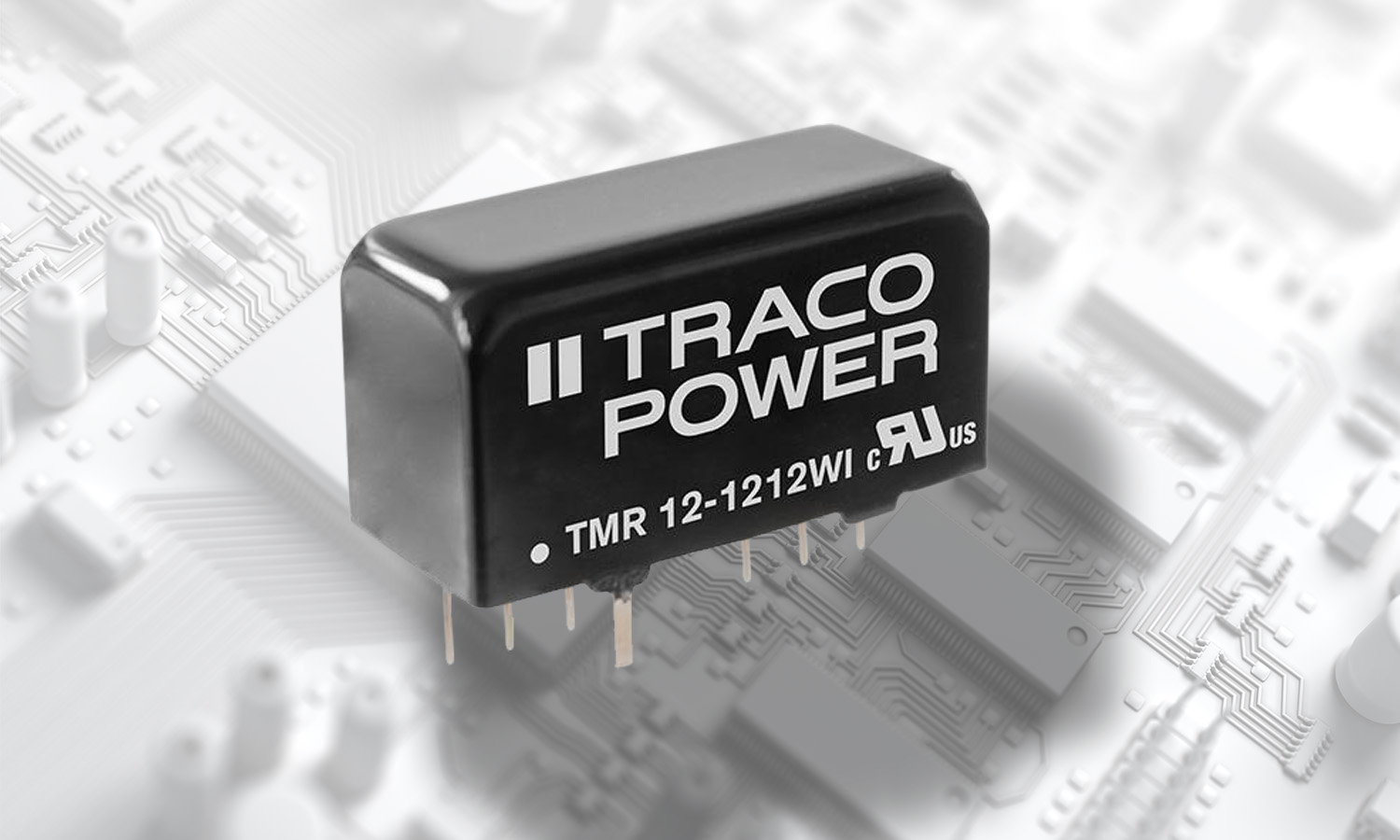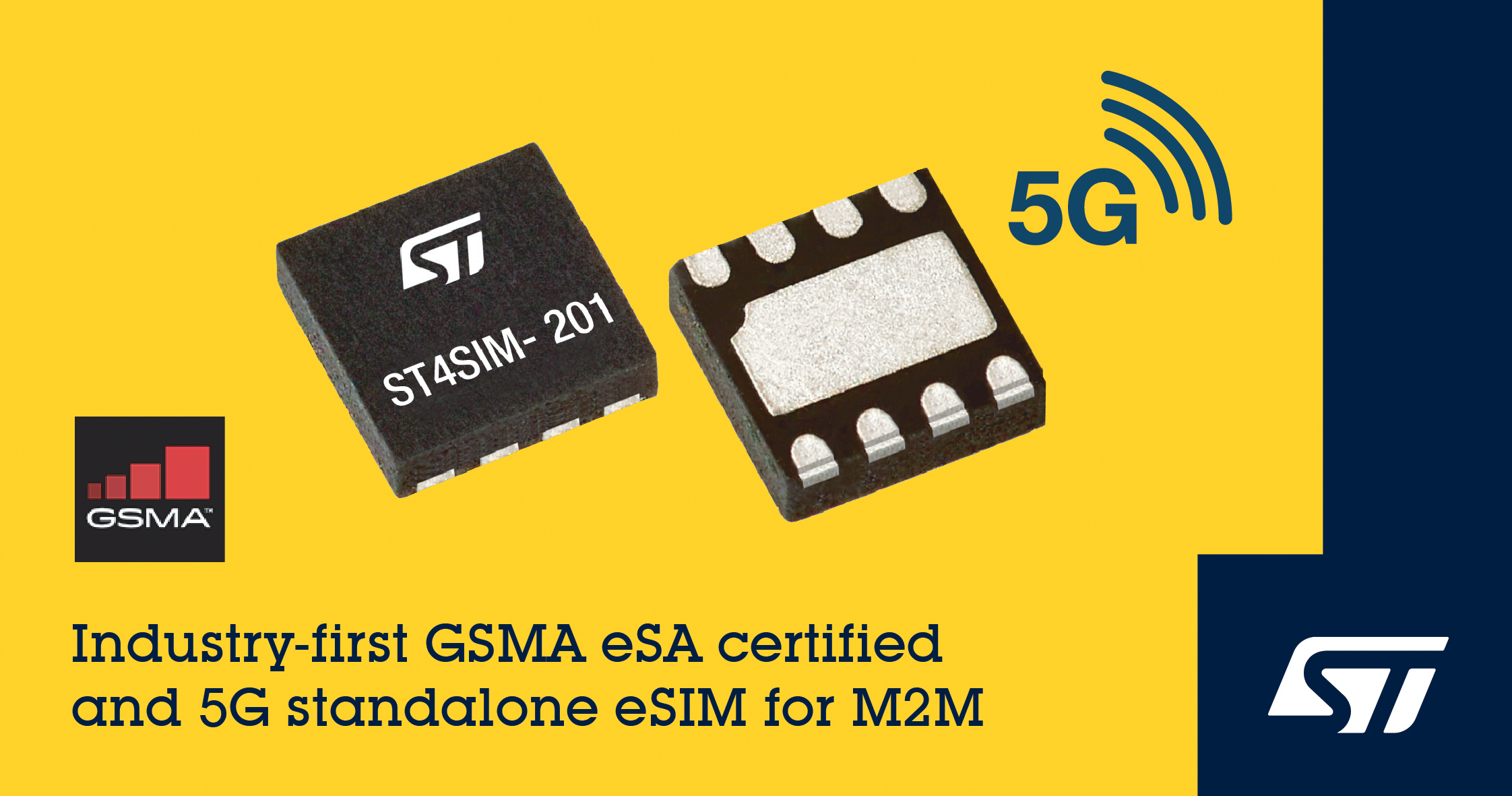# Advanced Power Metering in data centers

Author:
Kevin Huang, Analog Devices

Date
11/22/2014PDF
##### Digital communication techniques and intelligent power supplies enhance system management

The increasingly high cost of energy is pushing data centers and other related computing businesses toward finding intelligent strategies for power management at all levels. The implementation of such strategies requires an accurate collection of data for consumed power down to the power supply level. Today, digital communication techniques and intelligent power supplies make the task easier. Ppractical challenges still exist in implementing accurate power measuring because, with a few exceptions, power supplies are not measurement equipment.

Currently, AC power monitoring is implemented in some high-end systems, mainly by using dedicated ac power monitors and meters. However, in most cases, only the total rack power is monitored. Because most of these systems require a power factor correction (PFC) stage, where input current and voltage have to be measured by the control loop, it is logical to consider adding power metering within the PFC controller.

For example, the ADP1047/ADP1048 are digital PFC controllers with accurate input power metering capabilities built-in. They provide accurate measurement, such as input and output voltage, input current, and power. The information can be reported to the microcontroller of the power supply via the PMBus interface. By using a proper calibration algorithm, these errors can be mitigated. Two first-order, sigma-delta (Σ-Δ) analog-to-digital converters (ADCs) are used to sense the input voltage and input current. The sampling frequency of the ADCs is 1.6 MHz. True rms values are calculated at the end of each half ac line cycle by integrating the square of the instantaneous values.

Circuit setup and measurement

Figure 1 shows the ac power-metering interface. The measured point of the input current is the same as the one used for PFC current loop control. If the current sensing resistor is used, it measures the return current flowing through RSENSE after the main bridge rectifier. The input voltage is rectified through an auxiliary bridge rectifier, divided by the voltage divider (R1 and R2), and then fed into the ADP1047/ADP1048. The advantage of using the auxiliary bridge rectifier is that it can measure the input voltage accurately even during a soft start condition.

Click image to enlarge

Figure 1: AC power metering circuit configuration

This approach measures V and I within the PFC stage. In this case, the measurement is less challenging to perform and does not require isolation; however, it is subject to errors caused by the input bridge and EMI filter. These errors can be particularly significant at light load, when the currents in the EMI filter are comparable to the load currents. By using a proper calibration algorithm, these errors can be mitigated.

MEASUREMENT

Input voltage measurement

The input voltage is sensed through the voltage divider, R1 and R2, and the divided voltage is fed into the VAC ADC. The ADP1047 can internally calculate the input voltage value according to the VAC ADC reading and the value put in the 0xFE3B register, which is the input voltage resistor divider value. The input voltage is reported in linear format with a two-byte value. Y is an 11-bit, twos complement integer, and N is a 5-bit twos complement integer. Therefore, the real-world value is X = Y × 2N. The exponent of READ_VIN must be set in Register 0xFE39, Bits[5:3].

Input current measurement

The input current sensed signal is fed into the CS+ and CS− pins. The ADP1047/ADP1048 internally calculate the input current value according to the CS ADC reading and the input current sense ratio, IIN_GSENSE, which is set in the 0xFE3C register. The exponent of IIN_GSENSE is Bits[15:11] of the 0xFE3C register, and the mantissa is Bits[9:0] of the 0xFE3C register. There are nine exponent values available to program.

The input current is reported in linear format through the PMBus command. The exponent, Bits[10:6] of Register 0xFE39, must be programmed according to the maximum input current specification. To report IIN correctly, the following condition must be satisfied:

Input power measurement

The input power is reported with the PMBus command in linear format. There are eight exponent values available to set. The exponent, Bits[2:0] of Register 0xFE39, must be programmed according to the maximum input power specification. To report PIN correctly, the following condition must be satisfied:

For example, if the maximum PIN is 600 W, the exponent N should be larger than −2. To get the best resolution, the exponent N should be −1.

The averaging window is programmable from zero full line cycles to 4096 full line cycles using Bits[2:0] of Register 0xFE3A. At the end of each averaging period, the new value for average power is written to the READ_PIN register (Register 0x97) and is available to be read back through the interface until it is overwritten by the next averaged value at the end of the next averaging period (see Table 1).

Click image to enlarge

Table 1: Pin exponent options and maximum input power

TRIMMING

Input voltage digital trimming

The VAC ADC offset trim register trims the offset error of the input voltage, and the VAC ADC.

The CS ADC offset trim register trims the offset error of the PFC input current, and the CS ADC gain trim register trims the gain error of the PFC input current. Because the CS ADC input range has 500mV and 75 mV options, the trim registers are, respectively, available for each range selection.

Power metering digital trimming

The power metering offset trim for the low line input register is designed to correct the offset error to the input power measurement under the low line input. The power metering gain trim for the low line input register is designed to correct the gain error of the input power measurement under the low line input.

The power metering offset trim for the high line input register is designed to correct the offset error to the input power measurement under the high line input. The power metering gain trim for high line input register is designed to correct the gain error of the input power measurement under the high line input. These four registers are designed for customers to compensate power loss of the EMI filter and bridge rectifier.

Power metering digital trimming register definition

The following lists the register definitions for the power metering offset trim for the low line input register, the power metering gain trim for low line input register, the power metering offset trim for high line input register, and the power metering gain trim for high line input register.

For low line input,

When the power metering offset trim for low line input register, Bits[7:0] = 0x0, the offset range is 0; when the power metering offset trim for low line input register, Bits[7:0] = 0x7F, the offset is +6.2% of the full power; and when the power metering offset trim for low line input register, Bits[7:0] = 0xFF, the offset is −6.2% of the full power

When the power metering gain trim for low line input register, Bits[7:0] = 0x0, the gain error is zero; when the power metering gain trim for low line input register, Bits[7:0] = 0xFF, the gain error is −6.2%; and when power metering gain trim for low line input register, Bits[7:0] = 0x7F, the gain error is +6.2%.

For the high line input,

When the power metering offset trim for high line input register, Bits[7:0] = 0x0, the offset range is 0; when the power metering offset trim for high line input register, Bits[7:0] = 0x7F, the offset is +6.2% of the full power; and when the power metering offset trim for high line input register, Bits[7:0] = 0xFF, the offset is −6.2% of the full power.

When the power metering gain trim for high line input register, Bits[7:0] = 0x0, the gain error is zero; when the power metering gain trim for high line input register, Bits[7:0] = 0xFF, the gain error is −6.2%; and the when power metering gain trim for high line input register, Bits[7:0] = 0x7F, the gain error is +6.2%.

Experimental result

Measurements were performed on a 300 W demonstration board implemented using the ADP1047. Figure 2 shows measured power and real power. The demonstration board is designed to operate at 100 kHz in CCM, and the output voltage is regulated at 385 V. Figure 3 shows the measured power error compared with the commercial power meter under a wide input range with an ideal input source. The test is done at room temperature at 25°C.

Click image to enlarge

Figure 2: Measured power and real power

Click image to enlarge

Figure 3: Measured power error with ideal input AC source

Figure 4 through Figure 7 show the experimental results for nonideal sinusoidal ac line voltages at 25°C. In addition, they show the benefits of true rms power measurements so that the accuracy can be maintained even with nonideal ac line voltages. Note that the rectified input voltage for the input ac source shown in Figure 6 is approximately similar to the dc input voltage.

Click image to enlarge

Figure 4: Nonideal input AC source 1 waveform

Click image to enlarge

Figure 5: Measured power error with nonideal input AC source

Click image to enlarge

Figure 6: Nonideal input AC source 2 waveform

Click image to enlarge

Figure 7: Measured power over error with nonideal input AC source 2

### RELATED#### 12W DC/DC Converter in SIP-8 Package w/ 4:1 Input Range

Jun 30,2022#### 5G M2M Embedded SIMs Certified to Latest Industry Standards

Jun 15,2022#### System on Chip Features Flexibility, Low Power, and High-Performance Connectivity

Jun 14,2022May 31,2022